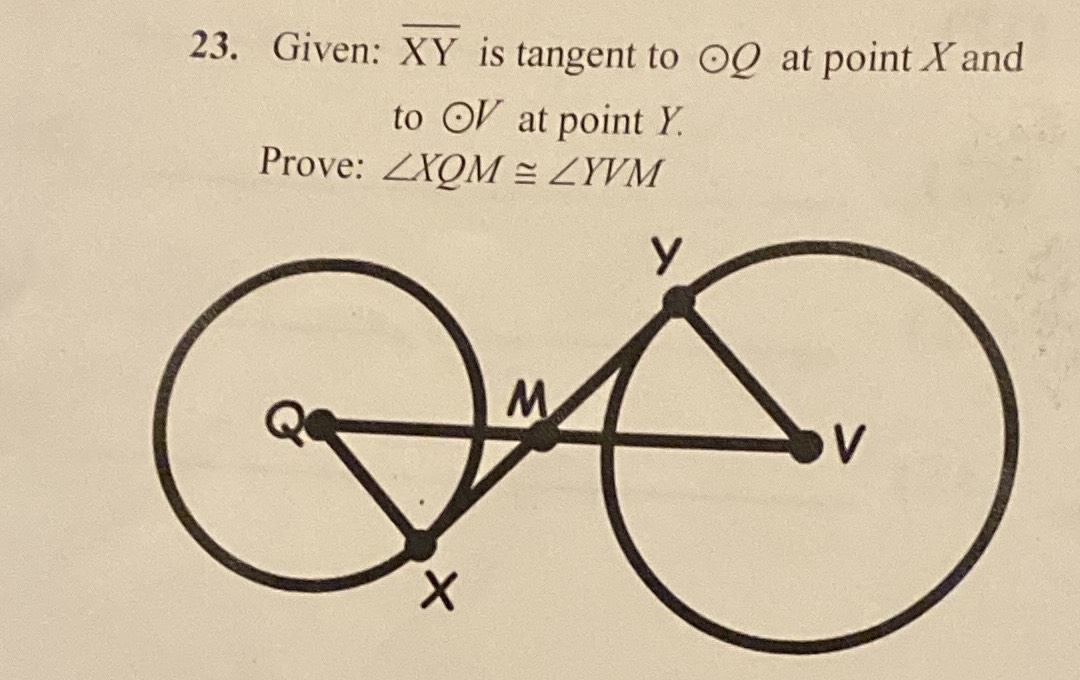¿Todavía tienes preguntas de matemáticas?

Pregunte a nuestros tutores expertos
Geometry
QuestionGiven: $$\overline{\mathrm{XY}}$$ is tangent to $$\odot\mathrm{Q}$$ at point $$\mathrm{X}$$ and to $$\odot\text{V}$$ at point $$\mathrm{Y}$$. Prove: $$\angle\mathrm{XQM}\cong\angle\mathrm{YVM}$$

$$\angle\mathrm{QXM}\cong\angle\mathrm{MYV}=90^{\circ}$$
$$\angle\mathrm{QMX}\cong\angle\mathrm{YMV}$$
$$\angle\mathrm{XQM}\cong\angle\mathrm{YVM}$$